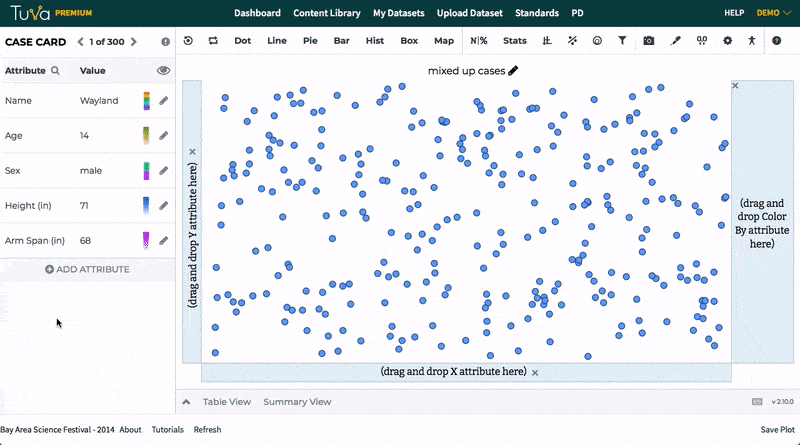# Tuva for Math

Tuva provides students with rigorous opportunities to learn foundational mathematics and statistics concepts by doing math — engaging in solving problems in authentic, real-world contexts. Whether you use Common Core State Standards, or your state’s own math standards, we have curated a library of content that aims to bring your standards to life.

## Why use Data to Teach Mathematics?

Using data to support mathematics instruction lends real world context to abstract mathematical concepts, and gives students the opportunity to apply their content knowledge in engaging ways. Rather than focusing on the procedural aspects of math instruction, the use of data allows teachers and students to live in the application.

 Standards for Mathematical Practice What role does data play in this practice? Make sense of problems and persevere in solving them Understand the meaning of a variable or coefficient in context using a dataset Experiment with manipulating data in order to answer a question appropriately Reason abstractly and quantitatively Investigate relationships between or among variables Continually ask “do these data make sense?” or “what are the limitations of these data?” Start with real-world applications to then decontextualize and understand abstract principles Construct viable arguments and critique the reasoning of others Clarify how patterns support or refute a claim Convey what a graph shows as reasoning for the claim Account for patterns in data as part of the claim. Write about data in descriptions, explanations, and interpretations Model with mathematics Use mathematical functions (e.g., frequencies, percentages, ratios, proportions, rates) to summarize and make predictions Describe a real world dataset in terms of statistical attributes (e.g., spread, shape, and center) Draw from existing data to make predictions based on probability Use graphical representations to model relationships between variables Use appropriate tools strategically Visualize and present data graphically, and decide which representation is best Look for errors or limitations of a model or tool in describing a real world issue Collect and analyze data as a part of planning and carrying out investigations Understand the purpose of calculators and data analysis tools Attend to precision Accurately communicate the meaning of an attribute, variable, coefficient, or solution given real world data Look for and make use of structure Sort and categorize data based on known attributes Look for and express regularity in repeated reasoning Search for regularity or trends in data through exploration Build an function or equation to fit and dataset and explore the fitness of that line

## Tuva for Elementary School Math

Students in grades 3-5 use Tuva to explore visual representations of data to order, classify, and make estimations. The dynamic plotting of data allows students to visualize the relationships between dot plots, bar charts, and pictographs, and aid students in their beginning understanding of how to choose the best method to represent a set of data.

Students also begin to use data to solve problems that include fractions and units of measure. Tuva lessons allow students to locate these concepts in authentic, real-world scenarios, and provides the pedagogical scaffolding to guide students toward conceptual understanding.

Choosing the best graph using Tuva
Tuva allows elementary students to explore various graph types and solidify their understanding of each. Students can quickly change between a dot plot, bar chart, or histogram and see how data are represented differently on each graph. They can also sort data and color code data to easily draw conclusions.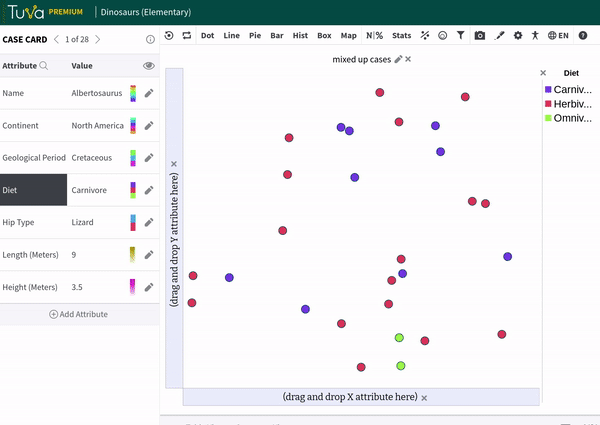## Tuva for Middle School Math

Student in Grades 6-8 use Tuva to explore distributions of data to better understand variability and central tendency. The dynamic plotting of data allows students to visualize the relationships between dot plots, box plots, and histograms and to understand the impact of outliers on measures of center and spread.

Students also construct bivariate plots to analyze patterns of association between two quantities and begin to create functions to model those relationships. Students are engaged by both the visual aspects of Tuva as well as the many different contexts of the datasets that allow them to contextualize the math concepts that they are learning.

Measures of Center - Mean and Median

Tuva allows middle school students to visualize challenging new concepts like how the mean and median are affected by extreme values in a data set and the impact of removing those data points. Students can quickly change between a dot plot and box plot to see how the measures of center are represented differently on each graph, allowing students to build more connections about these concepts.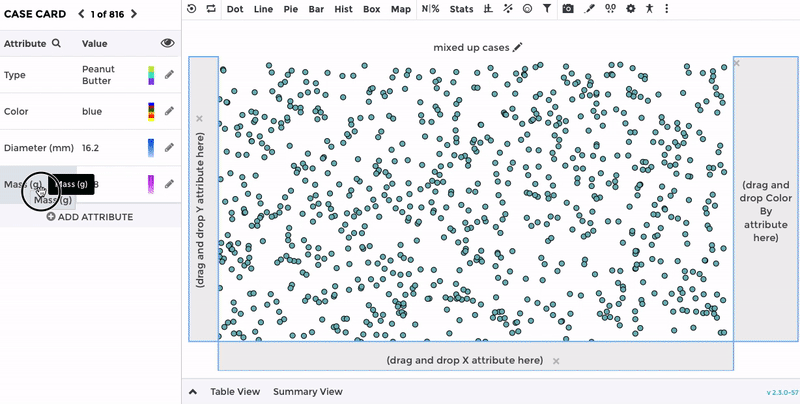## Tuva for High School Math

Students in high school use Tuva to investigate key concepts of statistics related to shape, center, and spread of distributions. Through the rich real-world contexts of Tuva datasets, students are able to examine key features of distributions in order to draw valid conclusions.

The dynamic nature of Tuva allows students to quickly compare distributions both visually and numerically using dot plots, box plots,and histograms. Additionally, high school students use Tuva to build models for bivariate data that are linear or nonlinear. The modeling features of Tuva allow students to either construct a function from scratch to fit a set of data or to compute a regression model of best fit. Students are then able to view summary statistic parameters for the model and use those to make predictions based on the context of the dataset they are working with in order to answer real-world problems.

Standard Deviation

Standard deviation is a challenging topic for students. Tuva can help students conceptually understand standard deviation through visual representation. Students use a reference lines to inspect the amount of overlap of two distributions in terms of the standard deviation, and use that relationship to draw conclusions. By seeing the standard deviation visually and immediately putting it into a real-world context, students have a much better understanding of this powerful statistical concept.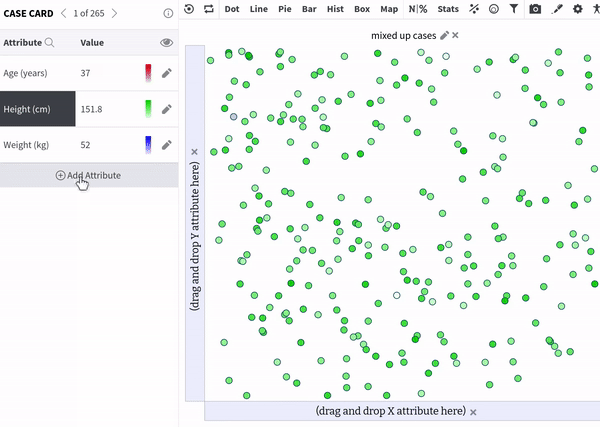Creating Linear & Nonlinear Models

Students use Tuva to dynamically build functions to model data in context. Students can see the impact of changing the coefficient of a quadratic model as they attempt to fit their function to a dataset. Tuva's modeling features strengthen student's conceptual understanding of the parameters of a function, while also highlighting the purpose of building a mathematical model in order to make predictions about real-world scenario.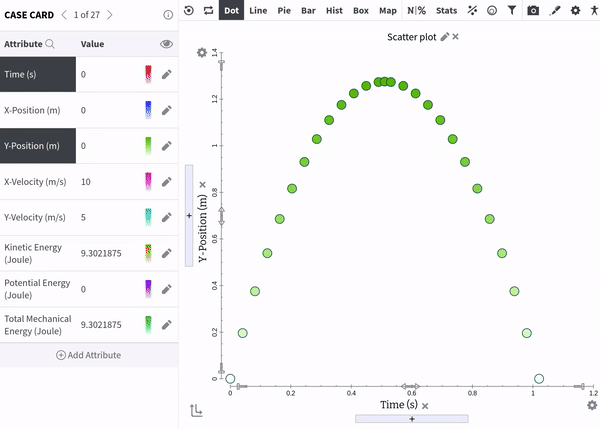## Tuva for AP Statistics

Tuva enhances the AP Statistics curriculum by giving students opportunities to visualize key concepts of variability, distribution shapes, and patterns of association for bivariate data. Students investigate engaging real-world datasets and use statistical concepts to make predictions and draw conclusions in context. Students can also use Random Sampling to generate a sampling distribution in order to explore the key concepts of the Central Limit Theorem.

Tuva's visual interface is ideal for students to understand the fundamental concepts of statistics that are need to progress in AP Statistics. Students can use Tuva to explore key concepts such as the Empirical Rule for a normal distribution. Through the use of dividers, they can calculate the percentage of data points within a given range, and they can test out whether a distribution is approximately normal.